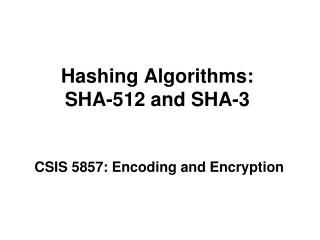DownloadDownload PresentationHashing Algorithms: SHA-512 and SHA-3

# Hashing Algorithms: SHA-512 and SHA-3

Télécharger la présentation## Hashing Algorithms: SHA-512 and SHA-3

- - - - - - - - - - - - - - - - - - - - - - - - - - - E N D - - - - - - - - - - - - - - - - - - - - - - - - - - -
##### Presentation Transcript

1. Hashing Algorithms: SHA-512 and SHA-3 CSIS 5857: Encoding and Encryption

2. Goals of Hashing Functions Hashing function must be “one way” • Easy to compute y = h(M) • Following must be computationally infeasible: • Given message M, find M´ such that h(M) = h(M´)(not vulnerable to preimage attack) • Finding any M1and M2 such that h(M1) = h(M1)(not vulnerable to collision attack)

3. Compression Functions What is a hash algorithm? • Function that compresses message of arbitrary length to m-bit digest Problem: • Difficult to assure collision resistance for arbitrary compression function f Message ofarbitrary size m-bit digest

4. Compression Functions • Easier to create function that compresses block of fixed sizek > m • Break message into blocks of fixed size • Apply compression function to each in some way f m-bit digest k-bit message

5. Iterated Hash Function Merkle-Damgard scheme

6. Iterated Hash Function • Compression function of form h(Mi, Hi-1) • M i = i th message block • Hi-1 = previous message digest • H0=initial vector known to sender, recipient • If f is collision resistant, so is entire algorithm n-bit message block M i f m-bit digest H i m-bit digest H i-1

7. Types of Hash Algorithms • Based on block ciphers • Rebuild existing cipher into compression function • Already has desirable properties of cryptographic hash • Confusion, diffusion • Example: Whirlpool • “Made from scratch” • Specifically designed for hashing • Often no clear structure for maximum confusion • Examples: • Message Digest (MD) by Ron Rivest (obsolete) • Secure Hash Algorithm (SHA-512, SHA-3)

8. Block Ciphers for Hashing • Rabin scheme • “Plaintext” = output of previous stage • “Key” = current message block • Potentially vulnerability: • Since encryption reversible, could use meet in middle attack • Work backwards from final message digest to find another M with same digest

9. Block Ciphers for Hashing • Miyaguchi-Preneel scheme (used by Whirlpool) • Output of each stage based on XOR of: • Output of encryption function • Output of previous stage • Current message • Prevents “meet in middle” attacks • Cannot work backwards through encryption functions without knowing input to previous stage

10. SHA-512 • Background: • Based on Merkle-Damgard scheme, Rivest MD5 • Ideas: • Large number of rounds (80) for maximum confusion • Heavy use of non-invertible functions • Combinations of rotation and XOR • Condition and majority functions • Appearance of “randomness” in initial vector • Designed for efficiency • All operations are very fast in hardware

11. SHA-512 • 512 bit message digest (secure against brute force attack) • Block size: 1024 bits • Digest broken down into 64 bit “words” called A – H

12. Word Expansion in SHA-512 • Block of 16 words expanded to 80 words • Used by 80-round compression function

13. Word Expansion in SHA-512 • Each word function of previous 4 words • Combined with XOR • Confusion added with rotation and shifting(not invertible) Right rotation i bits RotShift i-j-k Right rotation j bits  Left shift k bits (adding 0’s to end)

14. SHA-512 Initial Digest • Initial values of message digestH0 • Designed for appearance of “randomness” • Created from first 8 primes (2, 3, 5, 7, 11, 13, 17, 19) • Take square root • Take first 64 digits of fractional part

15. SHA-512 Compression Function • 80 rounds • Each creates new “intermediate” message digest • Final stage is sum (mod 264 ) of: • Initial round digest • Final round digest

16. SHA-512 Compression Function • Each roundi function of: • Previous message digest • Word Wi • Round “key” Kicreated from fractional parts of square root of first 80 prime numbers (like initial message digest values)

17. SHA-512 Round Structure • Blocks A – C and E – G shifted over one • No real effect, other than to make sure every block affected by more complex operations

18. SHA-512 Round Structure • New blocks A and E created as function of: • All previous blocks A – G • Round word Wiand round key Kiusing addition mod 264

19. SHA-512 Rotation Function • Next value of A, E based on previous value • Rotate right by 28, 34, and 39 bits • Combine with XOR to mix up bits

20. SHA-512 Majority Function • Majority function of (A, B, C): • Ith bit of result = 1 if at least 2 of ith bits of A, B, C = 10 otherwise • Example: A = 11001010B = 01101001C = 10011101majority = 11001001 • Idea: No way to reconstructA, B, C from majority

21. SHA-512 Conditional Function • Conditional function of (E, F, G): • Ith bit of result = ith bit of F if ith bit of E = 1 = ith bit of G oherwise • Like “If E then F else G” • Example: E = 11001010F = 01101001G = 10011101Conditional = 01011101 • Idea: No way to reconstructE, F, G from conditional

22. SHA-3 Competition • Open competition by NIST to design new standard for hashing algorithm • Same idea/goals as AES competition • Announced in 2008, Finalists chosen in 2010 • BLAKE • Grøstel (Lars Knudsen) • JH • Keccak (Joan Daemen) • Skein (Niels Ferguson, Bruce Schneier) • Winner: Keccak

23. SHA-3 S • Finalists: